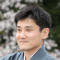Toshiyuki Syo
Principal Engineer

What is e-AI Translator?

A tool that converts learned AI algorithms created using an open source AI framework into C source code dedicated to inference.
The latest version of e-AI Translator, V2.1.0, was released on September 30, 2021.
In this blog, I will introduce the new function of V2.1.0 "8-bit quantization function by supporting TensorFlow Lite".

Bottleneck when processing AI on the MCU: memory resources

The needs are increasing that not only acquiring data but also making judgments by using AI algorithms on the system using MCUs and sensors.
If real-time performance is required for judgments, the MCU is the best platform for AI operating environment.
On the other hand, the problem of low memory resources is an obstacle to using AI algorithms on the MCU.
The AI algorithm is represented by a polynomial with many variables.
Therefore, compared to the conventional algorithm, it was difficult to allocate many AI parameters to a small amount of memory resources of MCU.
In recent years, the quantization of AI algorithms by TensorFlow Lite has become widespread to solve this memory resource problem.

ROM/RAM usage reduction effect of TensorFlow Lite 8bit quantization

First, please check how much the ROM / RAM usage reduction effect of the MCU by TensorFlow Lite 8bit quantization is.
As shown in the table below, the reduction effect varies depending on the structure of the model, but the maximum ROM / RAM reduction effect is 4.5 times.

Mechanism of 8-bit quantization and influence on inference accuracy

8-bit quantization changes the parameters and operations used in the AI model from the 32-bit float format to the 8-bit integer format.
As a result, it is possible to reduce the ROM usage for storing parameters and the RAM usage required for calculation.
On the other hand, there is a concern that the inference accuracy will decrease because the expressiveness of parameters and operations will decrease due to the reduction in the number of bits.
Then, please check how much the inference accuracy actually decreases.
As shown in the table below, there are differences depending on the model structure, but even the MobileNet v1 model, which has the largest accuracy difference, has a decrease in inference accuracy of about 1%.

8-bit quantization, which has a large effect of reducing ROM/RAM usage and has little decrease in accuracy, is an ideal function for MCUs.
Various networks can be executed on the MCU by using 8-bit quantization as shown in the figure below.

APPLICATIONS > Technologies > Embedded Artifical Inteligence (e-AI) > e-AI DevelopmentEnvironment & Downloads